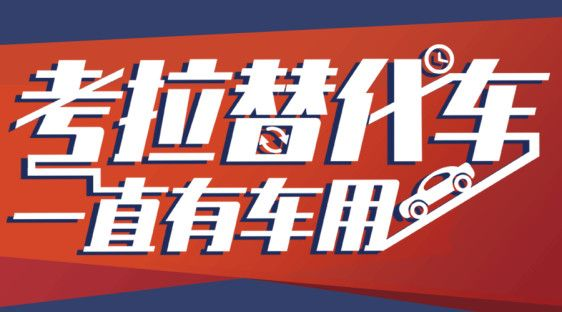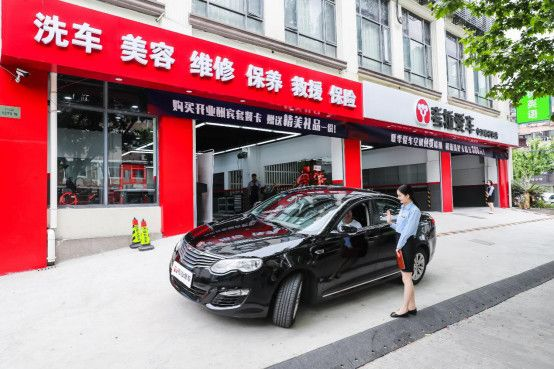## 考拉替代车登陆上海

2019年08月19日 11:07 来源：车行天下 超过：10555次关注

考拉替代车来了!

无缝衔接因维修造成的用车缺口，真正解决爱车维修期间无车可用的出行难题，考拉替代车，今日正式登陆上海，践行“考拉替代车，一直有车用”的服务理念，为行车生活保驾护航!考拉替代车，让行车生活零断档

爱车要在修车店一段时间，短时间内无车可用怎么办?考拉替代车，让行车生活零断档，凡在考拉爱车门店维修天数在两天或以上，车主即可享受考拉替代车服务，解决无车可用的尴尬状况。

考拉替代车，手续方便简单

用户选择替代车、提交资料、办理手续、验车、还车都可在考拉爱车门店直接办理，办理成功后，30——60分钟内，由专人送车到店，无需奔波，无需远距离取车，无需排队等待，店内专员对接，用户省心省力。

考拉替代车，便捷用户生活

在使用考拉替代车过程中，用户可根据自己使用情况，自由安排使用计划，当用户想提前归还替代车或遇到其它情况，也能通过客服热线轻松搞定，让用户行车生活更轻松。不仅如此，考拉替代车还支持异地还车，根据用户的实际使用需要，在同城不同门店、乃至省市之间灵活选择归还地，出行自由轻松，用车更方便。基于“1+5”覆盖体系，考拉替代车，一直有车用

考拉爱车门店连锁全国，合理布局，已在全国各地建立起“1+5”联动型覆盖体系，即围绕1家设备齐全、能做事故车维修、钣喷业务的综合店，开设N家分布在居民区周边的社区店。

通过整合资源、合理布局，离家近的社区店完全能胜任一般维修、日常保养、清洁美容，方便车主就近享受服务;当车辆需要比较复杂的维修时，车主亦可送车至社区店，再由考拉爱车员工将待维修车辆送至综合店完成，高效、方便，两不误。

与此同时，车主可直接在社区店选择使用考拉替代车，解决在车辆维修期间无车可用的问题。从爱车送修、取回到替代车租用、归还，都能在社区店一站式完成，0距离享受无缝隙的高品质专业服务，真正做到，考拉替代车，一直有车用!考拉替代车登陆上海，为上海消费者带来便民优质的服务。从此，即便遇上爱车暂时无法使用的情况，车主也无需改变用车习惯，考拉替代车将作为一个安心可靠的出行选择，让车主一直有车用!同时，在考拉爱车全方位的服务下，各位车主大可安心享受车生活，用车过程中出现任何烦心事，都有考拉爱车做您坚强的后盾。考拉爱车，让用车生活更轻松!

#### 相关文章

0-500 字已有评论 0条 查看评论>>

### 热门标签

﻿
• 快速找车
• 选择品牌
• 选择品牌
• A  奥迪
• A  阿斯顿·马丁
• A  阿尔法·罗密欧
• B  宝沃
• B  布加迪
• B  巴博斯
• B  保时捷
• B  宾利
• B  奔驰
• B  宝马
• B  本田
• B  别克
• B  标致
• B  比亚迪
• B  宝骏
• B  北汽制造
• B  北汽新能源
• B  北汽幻速
• B  北汽威旺
• B  北京汽车
• B  奔腾
• B  北汽绅宝
• C  长安
• C  长安商用
• C  长城
• C  昌河
• D  大众
• D  道奇
• D  DS
• D  东南
• D  东风风神
• D  东风风行
• D  东风小康
• D  东风风度
• D  东风
• F  福特
• F  丰田
• F  菲亚特
• F  法拉利
• F  福田
• F  福迪
• F  福汽启腾
• G  观致
• G  广汽传祺
• G  广汽吉奥
• G  GMC
• H  红旗
• H  汉腾汽车
• H  哈弗
• H  哈飞
• H  海格
• H  海马
• H  华颂
• H  黄海
• H  华泰
• H  恒天
• J  吉利汽车
• J  捷豹
• J  Jeep
• J  江淮
• J  江铃
• J  金杯
• J  九龙
• J  金旅
• K  凯翼
• K  凯迪拉克
• K  克莱斯勒
• K  科尼塞克
• K  卡威
• K  开瑞
• L  路虎
• L  林肯
• L  劳斯莱斯
• L  兰博基尼
• L  雷克萨斯
• L  铃木
• L  雷诺
• L  理念
• L  力帆
• L  莲花汽车
• L  猎豹
• L  路特斯
• L  陆风
• M  马自达
• M  MG
• M  MINI
• M  玛莎拉蒂
• M  摩根
• M  迈凯轮
• N  纳智捷
• O  欧宝
• O  讴歌
• O  欧朗
• Q  奇瑞
• Q  起亚
• Q  启辰
• R  日产
• R  荣威
• R  瑞麒
• S  三菱
• S  斯威汽车
• S  萨博
• S  smart
• S  斯柯达
• S  斯巴鲁
• S  思铭
• S  双龙
• S  上汽大通
• S  双环
• T  特斯拉
• T  腾势
• W  沃尔沃
• W  五菱汽车
• W  五十铃
• W  威兹曼
• W  威麟
• X  现代
• X  雪佛兰
• X  雪铁龙
• X  西雅特
• Y  一汽
• Y  英菲尼迪
• Y  英致
• Y  依维柯
• Y  野马汽车
• Y  永源
• Z  众泰
• Z  中华
• Z  中兴
• Z  知豆
• 选择车系
• 选择车系
• 车型对比
• 选择品牌
• 选择品牌
• A  奥迪
• A  阿斯顿·马丁
• A  阿尔法·罗密欧
• B  宝沃
• B  布加迪
• B  巴博斯
• B  保时捷
• B  宾利
• B  奔驰
• B  宝马
• B  本田
• B  别克
• B  标致
• B  比亚迪
• B  宝骏
• B  北汽制造
• B  北汽新能源
• B  北汽幻速
• B  北汽威旺
• B  北京汽车
• B  奔腾
• B  北汽绅宝
• C  长安
• C  长安商用
• C  长城
• C  昌河
• D  大众
• D  道奇
• D  DS
• D  东南
• D  东风风神
• D  东风风行
• D  东风小康
• D  东风风度
• D  东风
• F  福特
• F  丰田
• F  菲亚特
• F  法拉利
• F  福田
• F  福迪
• F  福汽启腾
• G  观致
• G  广汽传祺
• G  广汽吉奥
• G  GMC
• H  红旗
• H  汉腾汽车
• H  哈弗
• H  哈飞
• H  海格
• H  海马
• H  华颂
• H  黄海
• H  华泰
• H  恒天
• J  吉利汽车
• J  捷豹
• J  Jeep
• J  江淮
• J  江铃
• J  金杯
• J  九龙
• J  金旅
• K  凯翼
• K  凯迪拉克
• K  克莱斯勒
• K  科尼塞克
• K  卡威
• K  开瑞
• L  路虎
• L  林肯
• L  劳斯莱斯
• L  兰博基尼
• L  雷克萨斯
• L  铃木
• L  雷诺
• L  理念
• L  力帆
• L  莲花汽车
• L  猎豹
• L  路特斯
• L  陆风
• M  马自达
• M  MG
• M  MINI
• M  玛莎拉蒂
• M  摩根
• M  迈凯轮
• N  纳智捷
• O  欧宝
• O  讴歌
• O  欧朗
• Q  奇瑞
• Q  起亚
• Q  启辰
• R  日产
• R  荣威
• R  瑞麒
• S  三菱
• S  斯威汽车
• S  萨博
• S  smart
• S  斯柯达
• S  斯巴鲁
• S  思铭
• S  双龙
• S  上汽大通
• S  双环
• T  特斯拉
• T  腾势
• W  沃尔沃
• W  五菱汽车
• W  五十铃
• W  威兹曼
• W  威麟
• X  现代
• X  雪佛兰
• X  雪铁龙
• X  西雅特
• Y  一汽
• Y  英菲尼迪
• Y  英致
• Y  依维柯
• Y  野马汽车
• Y  永源
• Z  众泰
• Z  中华
• Z  中兴
• Z  知豆
• 选择车系
• 选择车系
• 选择车型
• 选择车型
• 意见反馈